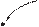### Create an Account

Home / Questions / A wire of length 20 cm vibrates in one direction in a constant magnetic field with a flux...

# A wire of length 20 cm vibrates in one direction in a constant magnetic field with a flux density of 01 T see Figure P1345 The position of the wire as a function of time is given by

A wire of length 20 cm vibrates in one direction

in a constant magnetic field with a flux density of

0.1 T; see Figure P13.45. The position of the wire as a function of time is given by x(t) = 0.1 sin 10m. Find the induced emf across the length of the wire as a

function of time.

+       –

v(t)

20 cm

X   X  X  X

 X X X X X X X X X X X e(t) X – X X X X X X X X X X X X X X X X X X X X

+Wire

X   X   X  X

Jun 18 2020 View more View LessSubscribe To Get Solution